International Journal of Mineral Processing and Extractive Metallurgy
Volume 1, Issue 4, September 2016, Pages: 26-32

The Management of Zinc Concentrate Acquisition in "Trepca"

Ahmet Haxhiaj1, Mehmet Deniz Turan2, Bersant Beka1

1Faculty of Geosciences, University of Mitrovca "Isa Boletini", Mitrovica, Republic of Kosovo

2Metallurgy and Materials Eng. Dept. Eng. Faculty, Firat University, Elazig, Turkey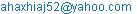(A. Haxhiaj)

Ahmet Haxhiaj, Mehmet DenizTuran, Bersant Beka. The Management of Zinc Concentrate Acquisition in "Trepca". International Journal of Mineral Processing and Extractive Metallurgy. Vol. 1, No. 4, 2016, pp. 26-32. doi: 10.11648/j.ijmpem.20160104.12

Received: August 24, 2016; Accepted: August 29, 2016; Published: September 26, 2016

Abstract: Based on the placement of zinc and its consumption in branches of industry we treat zinc composition of Kopaonik massif. Avulsion, and melting sphaleritetenuity. The paper in particular makes delivery of the flotation process. Treats bases reagents physic-chemical process. Also treated decanting, drying, filtering and storage of concentrates., Verification of the chemical composition of Zn concentrates with Zn, Pb, Bi, As, Sb, In, Au, Ag, etc. The special is that we massif Kopaonik composition ratio of Pb, Zn is 1.4: 1.0 and zinc flotation is over I opted to go with the Pb concentrate more than is allowed. We are strongly concentrated in the maximum amount of Zn that goes with the Pb concentrates and tailings. As such work has given first effects of these parameters in optimizing the positive effects of the flotation process in "Trepca".

Keywords: Mineral Reagents, Grinding, Flotation, Concentrate Zn, Sterile

Contents

1. Introduction

Managing the amount of zinc concentrates. The amount of zinc in concentrates Zn and we sterile. Zn amount of residues in the flotation process in "Stanterg" It is important to separate. The process is complex and necessary in the technological process of obtaining zinc. The article addresses: Equipment Components, Components and Operation Components Chemistry. We dosed mineral flotation process sulfur which has this chemical compound 3.85%.≤Pb ≥ 3.17% and 2.54%≤Zn≤3.425% and 51.6g/t-1≤Ag≤59.2g/t-1. Minerals such as enrichment prefer selective extraction of the flotation process. Composition of zinc waste and losses with residue is complex and depends on many factors. Managing the amount of the Zn concentrate and zinc losses with residue is object of study in this paper. Which refers to the process parameters of flotation technology. Parameters that influence the losses of zinc residues are: load balance, load temperature, chemical composition crushing and flotation load and cooling the load. Analysis of industrial flotation process, chemical analysis products during the flotation concentrates of zinc benefit made to achieve positive effects, technical and technological and economic process of flotation.

2. Froth Flotation-Fundamental Principles

Froth flotation is a highly versatile method for physically separating particles based on differences in the ability of air bubbles to selectively adhere to specific mineral surfaces in a mineral/water slurry. The particles with attached air bubbles are then carried to the surface and removed, while the particles that remain completely wetted stay in the liquid phase. Froth flotation can be adapted to a broad range of mineral separations, as it is possible to use chemical

Treatments to selectively alter mineral surfaces so that they have the necessary properties for the separation. It is currently in use for many diverse applications, with a few examples being: separating sulfide minerals from silica gangue (and from other sulfide minerals); separating potassium chloride (sulvite) from sodium chloride (halite); separating coal from ash-forming minerals; removing silicate minerals from iron ores; separating phosphate minerals from silicates; and even non-mineral applications such as de-inking recycled newsprint. It is particularly useful for processing fine-grained ores that are not amenable to conventional gravity concentration.

2.1. Particle/Bubble Contact

Once the particles are rendered hydrophobic, they must be brought in contact with gas bubbles so that the bubbles can attach to the surface. If the bubbles and surfaces never come in contact, then no lotation can occur. Contact between particles and bubbles can be accomplished in a flotation cell such as the one shown schematically in Figure 5.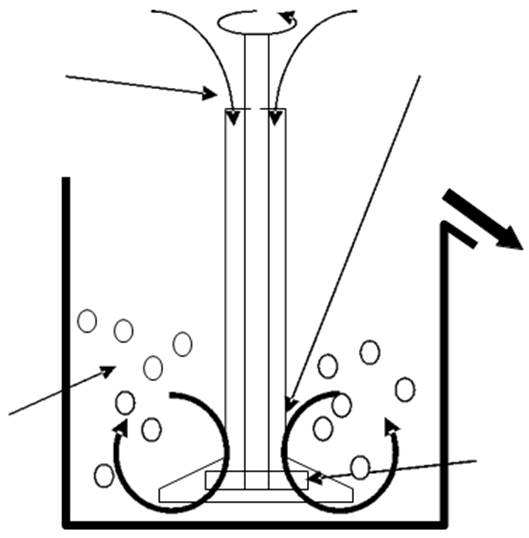Fig. 1. Simplified schematic of a conventional flotation cell. The rotor draws slurry through the stator and expels it to the sides, creating a suction that draws air down the shaft of the stator. The air is then dispersed as bubbles through the slurry, and comes in contact with particles in the slurry that is drawn through the stator. Particle/bubble collision is affected by the relative sizes of the particles. If the bubbles are large relative to the particles, then fluid flowing around the bubbles can sweep the particles past without coming in contact. It is therefore best if the bubble diameter is comparable to the particle diameter in order to ensure good particle/bubble contact.

2.2. Conventional Cells

Conventional flotation cells consist of a tank with an agitator designed to disperse air into the slurry, as was previously shown schematically in Figure 5. These are relatively simple machines, with ample pportunity for particles to be carried into the froth along with the water making up the bubble films (entrainment), or for hydrophobic particles to break free from the froth and be removed along with the hydrophilic particles. It is therefore common for conventional flotation cells to be assembled in a multi-stage circuit, with "rougher", "cleaner", and "scavenger" cells, which can be arranged in configurations such as the one shown in Figure 2.2.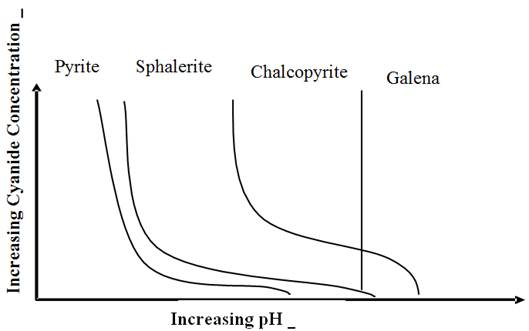Fig. 2. One possible configuration for a Rougher/Cleaner/Scavenger flotation circuit.

2.3. Reagents

The properties of raw mineral mixtures suspended in plain water are rarely suitable for froth flotation. Chemicals are needed both to control the relative hydrophilicities of the particles, and to maintain the proper froth characteristics. There are therefore many different reagents involved in the froth flotation process, with the selection of reagents depending on the specific mineral mixtures being treated.

Anionic Collectors for Sulfide Minerals

The most common collectors for sulfide minerals are the sulfhydryl collectors, such as the various xanthates and dithiophosphates. Xanthates are most commonly used, and have structures similar to what is shown in Figure 8. Xanthates are highly selective collectors for sulfide minerals, as they chemically react with the sulfide surfaces and do not have any affinity for the common non-sulfide gangue minerals. Other highly-selective collectors for use with sulfide minerals, such as ithiophosphates, have somewhat different adsorption behavior and so can be used for some separations that are difficult using xanthates.Fig. 3. Structure of a typical xanthate collector (ethyl xanthate). The OCSS- group attaches irreversibly to the sulfide mineral surface. Using xanthates with longer hydrocarbon chains tends to increase the degree ofhydrophobicity when they adsorb onto the surface.

3. Management of Mineral Resources of Zinc

Spot sources of Zn ore deposits are composed that besides Zn minerals also contain significant amounts of minerals t Pb. So in most cases when it comes to mean Zn ore of lead and zinc ore. Smaller quantities are also present metal ore minerals such as Au and Ag minerals, minerals Fe, Cu minerals minerals Bi etc.

Table 1. Presents the chemical composition of lead-zinc ores in the Kopaoniku Mountains massif.

 Type of ore %Pb %Zn g/t Ag %Fe %Cu %S %SiO2 1 5.5 13.0 - 9.4 - - 18.0 2 8.5 13.8 - 1.8 1.0 - 20.0 3 6.0 13.1 - - - 16.0 19.0 4 4.0 2.8 60 - - - - 5 2.8 2.1 51 - - - - 6 3.7 2.4 52 - - - -

Trepca Mines has modern infrastructure by contemporary model of underground mining. I have three wells developed 12 horizons and the horizons of the last three are developed ramps that enable the application of modern mechanisms for preparing the ore.

Mines with its infrastructure with modern methods and has the capacity to fill the mineral exploitation of 500000 to 650000 tons in the year.

Quality of Sphalerite, with sulfur Zinc forms only one component which is found in nature in the form of pure ore as Sphaleritewith shiny blue color. Temperature of fusing his boiling temperature 491°C 907°C. In the absence of air sphalerite begins to sublimate at about 750°C. Sphalerite belongs to the group of components that evaporate easily and this feature of it is the main cause of loss of zinc during its processing. Spot zinc resources in Kosovo lie in the so called Trepca metallurgical belt. Which lies in the northeastern part of Kosovo starting from Leposavic to Gjilan. Height of the belt is on average 80 km and the width of the belt is 30 km.

Table 2. Present the total reserves and zinc ore.

 The origin Ore(ton) %Zn Metal of Zn in(ton) Stan Terg 35081000 2.54 8910574 CerrnacBBGom 7544227 3.425 2583897 Kompleksin ArtanaCB 16837227 2.40 6734890 Total 58662569 2.788 16308194.18

4. Flotation Process

Ore by Stanterg mine for the concentrator carried by train.

The flotation process comprises three stages of the important matters that are:

1.  Grinding and Tenuity

2.  Classification of sizes, and

3.  Conditioning

Grinding and finalize tenuity consider when ore I legislated by mines with the size 400mm tenuity in the size 0.074 μm. The broken ore to 0.6 mm along with reagents weighty 74 t/h dosed mill for final tenuity. Measure/Pulp of tenuity at 0.074 μm diameter particles from the milling funnel Φ = 100 mm transferred to the zinc conditioner.

The mineral flotation process is based on physical-chemical virtues of each mineral phase and the laws of their interaction. The basic goal of the flotation process is the separation of compounds useful selective by others. To achieve a goal t is necessary for the mining of aore ingredient remain in limbo in the aquatic environment. Being at the same time also distributed with bubbles airing sent in this environment. Under conditions where such a mixture is put into motion the dynamics of rigid phases, juice, flotationenvironment gases. Selective separation occurs minerals where some of them I attached bubbles and the rest remains with water.

Bubbles/Aggregate [Froth] have -bubbles and mineral particles less than density aqueous medium to rise to the surface and pulp forming the flotation product {Concentrate process). The mineral particles interact with air bubbles is possible in those cases where these virtues Surface of hydrophobic particles have a natural ability or created by the activity and impact of the reagents used in the flotation process.

Table 3. Is presented reagent type and quantity used in the flotation of zinc ore.

 Name of reagent Place Quantity we cm3 mn-1 or ml SodiumCianidi (NaCN) grinder 400 Sulphate I Zinc (ZnSO4) grinder 700 Soda (Na2CO3) grinder 3 kg t-1 Industrial water grinder 1.5 m3 min-1

In general, divided into three groups reagents. Collector reagent, reagent adjustment, went forming bubbling/reagent. And there are the experience use is industrial art.

The flotation process zinc ore has three main processes. Basic flotation, Controller flotation and Scavenger flotation.

Basic flotation and Controller flotation is performed pneumatic machines with impeller type "Denver" series.We are placed 12 cells, each cell has a volume V = 2.83 m3.

Scavenger flotation done in machines with impeller and mechanical ventilation. The car has 14 cells, each cell has a volume V = 1.1m3. The whole technological process l flotation that shown in Fig. 12.

Tenuitymass of 0.074 μm as digestion dosed based flotation cell in car 12 and the product surface-with the help of the choir carried shovels for car number 8 of flotation cleaners. While the lower part is carried in the entrance-current controller for ri-flotation to flotation. The flotation product controller is managed as follows. The amount of the 6 cells before cell number 4 is transmitted in the flotation cleaners as final product (concentrate) product and 6 other flotation cells return to the air conditioner controller While the lower steps of the flotation cell controller 6 goes as sterile.

5. Performance Calculations

There is no universal method for expressing the effectiveness of a separation, but there areseveral methods that are useful for examining froth flotation processes:

(a).  Ratio of Concentration, the weight of the feed relative to the weight of the concentrate.

The Ratio of Concentration is F/C, where F is the total weight of the feed and C is the total weight of the concentrate. One limitation with this calculation is that it uses the weights of the feed and concentrate. While this data is available in laboratory experiments, in the plant it is likely that the ore is not weighed and only assays will be available. However, it is possible to express the ratio of concentration in terms of ore assays.

Starting with the mass balance equations, and the definition of the ratio of concentration:

F = C + T, Ff = Cc + Tt, Ratio of Concentration = F/C where F, C, and T are the% weights of the feed, concentrate, and tailings, respectively; and f, c, and t are the assays of the feed, concentrate, and tailings. We now need to eliminate T from these equations so that we can solve for F/C:

Ff = Cc + Tt, and multiplying (F = C + T) by t gives us:

Ft = Ct + Tt, so subtracting this equation from the previous eliminates T and gives:

F (f - t) = C(c - t), and rearranging produces the equation for the ratio of concentration:

F/C = (c – t)/(f – t)1

(b).  % Metal Recovery, or percentage of the metal in the original feed that is recovered in the concentrate. This can be calculated using weights and assays, as (Cc)/(Ff)·100. Or,since C/F = (f – t)/(c – t), the% Metal Recovery can be calculated from assays alone using 100(C/F) (f – t)/(c –t).

(c).   % Metal Loss is the opposite of the% Metal Recovery, and represents the material lost to the tailings. It can be calculated simply by subtracting the% Metal Recovery from 100%.

(d).  % Weight Recovery is essentially the inverse of the ratio of concentration, and equals100·C/F = 100· (f – t)/(c – t).

(e).   Enrichment Ratio is calculated directly from assays as c/f, weights are not involved in the calculation.

The main parameters of the technological process of flotation lead company "Trepca"

F = 150 t h-1 was dosed for flotation loads.

C - The weight of the concentrate which depends on technological settings t flotation process.

T –Losses weight,sterility that depends on the quality of mineral and in technological flotation process.

f - Percentage of metal in ore.

c - Percentage of metal in concentrate that depends on the quality of technological flotation process.

t - Percentage of metal in sterile that depend on the quality oftechnological flotation process.

Alternative I, the calculation of the quantity of zinc concentrate, percentage of Zn in the Zn concentrate and Zn losses with residue when Zn ore containing 2.54%.

a.  The amount of the Zn concentration is calculated with mathematical expression.

F/C = (c – t)/(f – t)

F= 150 t

Laboratory analysis of ore, concentrate and sterile made in the Laboratory of Trepça Company dated 18.06.2013:

f= 2.54% Zn

c = 46.33% Zn

t = 0.33% Zn

C= F{ f-t)/(c-t)= 150 (2.54- 0.33)/(46.33-0.33) =7.2065t h-1

C = 7.2065 t h-1

b.  The amount of Zn in the Zn concentrate calculated with mathematical expression.

CZn = C x c th-1

CZn = 7.2065 x 0.463 th-1

CZn = 3.3366 t h-1

c.   The amount of Zn in the waste that has gone with the Pb with pirot concentrate andsterile,calculated with mathematical expression.

Cresidue = CZn mineral—CZnt h-1

Cresidue=3.81 – 3.3366 t h-1

Cresidue= 0.4734t h-1

d.  Percentage Weight Recovery is essentially the inverse of the ratio of concentration, and equals Mathematical expression.

100·C/F = 100·(f – t)/(c – t).

100·C/F - 100·(f – t)/(c – t)= C/F -(f – t)/(c – t) = 7.2065/150- (2.54-0.33)/(46.33-0.33) = 0.0000047% Zn Is the load concentration of Zn.

e.   The ore enrichment, loads calculated with mathematical expression.

c/f%

46.33/2.54 = 18.2401%is enrichment of solid ore.

f.   weight of residues is calculated with mathematical expression.

F = C + T t h-1

T= F - Ct h-1

T = 150 - 7.2065 = 142.7935 t h-1

142.7935 t h-1Is the amount of waste which in itself contains Pb Concentrate, concentrate the pirotines and sterile.

g.   Zinc enrichment Report calculated with mathematical expression

Renrichment Zn =%ZnConcentrate/%Znore

Renrichment Zn = 46.33/2.54 = 18.240%

Renrichment Zn = 18.240%Is the enrichment of Zn during the flotation process

Alternative II, the calculation of the quantity of zinc concentrate, concentrate percentage of Zn in the Zn and Zn losses with residue when Zn ore containing 3.425%.

a.  The amount of the Zn concentrate calculated with mathematical expression.

F/C = (c – t)/(f – t)

F = 150 t

Laboratory analysis of ore, concentrate and sterile made in the Laboratory of Trepca Company dated 19.06.2013:

f= 3.425% Zn

c = 47.75% Zn

t =0.32%Pb

C= F{ f-t)/(c-t)= 150 (3.425- 0.32)/(47.75 - 0.32) = 9.758t h-1

C = 9.758t h-1

b.  The amount of Zn in the Zn concentrate calculated with mathematical expression.

CZn = C x c th-1

CZn = 9.758 x 0.477 th-1

CZn = 4.654 t h-1

c.   The amount of Zn in the waste that has gone with the Pb with pirot concentrate andsterile,calculated with mathematical expression.

C residue = CZn ore—CZnt h-1

C residue = 5.137 –4.654th-1

C residue = 0.482t h-1

d.  % Weight Recovery is essentially the inverse of the ratio of concentration, and equals mathematical expression.

100·C/F - 100·(f – t)/(c – t)= C/F - (f – t)/(c – t) = 9.758/150 - (3.425 – 0.32)/(47.75 – 0.32) = 0.00046%

0.00046%It Is ZN concentration of the load.

e.   The ore enrichment, loads calculated with mathematical expression.

c/f%

47.75/3.425 = 13.941%is enrichment of solid ore.

f.   weight of residues is calculated with mathematical expression.

F = C + T t h-1

T= F - Ct h-1

T = 150 – 9.758 = 140.242t h-1

140.242 t h-1 Is the amount of waste which in itself contains Pb Concentrate, concentrate the pirotines and sterile.

g.   Zinc enrichment Report calculated with mathematical expression

Renrichment Zn =%ZnConcentrate/%Znore

Renrichment Zn = 47.75/3.425 = 13.941%

Renrichment Zn = 4.993 = 13.941 Is the enrichment of Zn during the flotation process

Alternative III, the calculation of the quantity of zinc concentrate, concentrate percentage of Zn in the Zn and Zn losses with residue when Zn ore containing 2.40%.is taken by the Mines of Artane.

a.  The amount of the Zn concentration is calculated with mathematical expression.

F/C = (c – t)/(f – t)

F= 150 t

Laboratory analysis of ore, concentrate and sterile made in the Laboratory of Trepça Company dated 20.06.2013:

f= 2.46% Zn

c = 48.32% Zn

t = 0.23% ZN

C= F{ f-t)/(c-t)= 150 (2.46 - 0.23)/(48.32 - 0.23) =6.955t h-1

C = 6.955t h-1

b.  The amount of Zn in the Zn concentrate calculated with mathematical expression.

CZn = C x c th-1

CZn = 6.955 x 0.483 th-1

CZn = 3.259 t h-1

c.   The amount of Zn in the waste that has gone with the Pb with pirot concentrate andsterile,calculated with mathematical expression.

Cresidue = CZn mineral—CZnt h-1

Cresidue=3.69– 3.259t h-1

Cresidue= 0.431t h-1

d.  Percentage Weight Recovery is essentially the inverse of the ratio of concentration, and equals Mathematical expression.

100·C/F - 100·(f – t)/(c – t)= C/F - (f – t)/(c – t) = 6.955/150 - (2.46 – 0.23)/(48.32 – 0.23) = 0.00001%

0.00001%Zn Is the load concentration of Zn.

e.   The ore enrichment, loads calculated with mathematical expression.

c/f%

48.32/2.46 = 19.642% is enrichment of solid ore.

f.   weight of residues is calculated with mathematical expression.

F = C + T th-1

T= F - Ct h-1

T = 150 – 6.955 = 143.045t h-1

143.045 t h-1 Is the amount of waste which in itself contains Pb Concentrate, concentrate the proteins and sterile.

g.   Zinc enrichment Report calculated with mathematical expression

Renrichment Zn =%ZnConcentrate/%Znore

Renrichment Zn = 48.32/2.46 = 19.642%

Renrichment Zn = 19.642%Is the enrichment of Zn during the flotation process

Table 4. Presents amount of Zn in the mineral, amount of concentration of Zn, amount of Zn in the concentrate, and amount of Zn as residues for:Alt. I, II, III.

 Alternatives Amount of Zn in the mineralth-1 Amount of concentration of Zn t h-1 Amount of Zn in the concentrate th-1 Amount of Zn as residues t h-1 Alt.I 3.81 7.206 3.336 0.473 Alt.II 5.137 9.758 4.654 0.482 Alt.III 3.60 6.955 3.259 0.431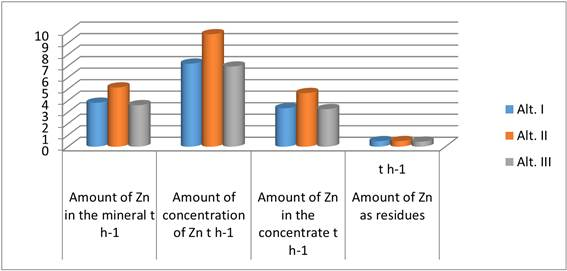Fig. 4. Presents amount of Zn in the mineral, amount of concentration of Zn, amount of Zn in the concentrate, and amount of Zn as residues for:Alt. I, II, III.

Table 5. t represents enrichment of ore in Zn Alt. I, II, III.

 Alternativ The enrichment of ore% Enrichment of Zn% Alt. I 18.2401 18.240 Alt. II 13.941 13.941 Alt. III 19.642 19.642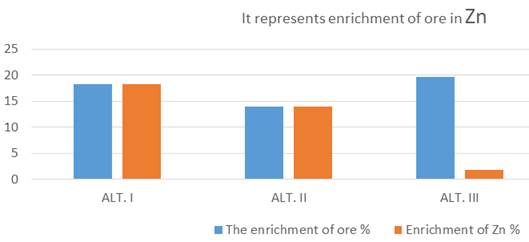Fig. 5. Represents enrichment of ore in Zn Alt.I, II, III.

6. The Discussion of Results

With industrial works technological process of flotation in "Trepça" and I treat laboratory analysis main parameters which have powerof functional/management in the process of flotation. WeAlt.I II III in industrial process and laboratory analysis Zn ore article deals with percentage, "Alt. I" 2.54% Znand the percentage"Alt. II" 3.425%Zn,."Alt. III" 2.40% Zn.

And note that:

The amount of Zn in the ore is: Alt.I=3.81 t h-1. Alt.II= 5.137 t h-1. Alt.III=3.60 t h-1.The amount of the Zn concentrate and the amount of Zn in concentratein Zn per "Alt.II" has increased to Alt I with rat C = 2.5515 t h-1 while on "Alt. III" with rat C = 2.803 t h-1. For the amount of Zn in the concentrate have increased "Alt. I" C(concentrate)Zn.The value = 1.3174 t h-1 and C(concentrate)Zn "Alt. III" = 1.395 t h-1.

The amount of Zn in the residues for "Alt.. II" has increased to "Alt. I". The value 0086 t h-1worthwhile to "Alt.III" with 0.051 t h-1

Enrichment of ore per "Alt.II" has a value decreasing to "Alt.I" 4.298 t h-1 is worth while to "Alt.III" 5.7 t h-1

The quantity of waste per Alt. II has a rest "Alt.I" shutdown in value 2.551 t h-1 while spills "Alt.III" value -1 2803t h-1.

The enrichment of Zn during the flotation process for "Alt. II" has a decreasing value to 4.298% "Alt. I" while rest "Alt.III" value 5.7%.

These are the parameters of technological process of flotation which identify the better management or not better technological process of the Zn ore flotation in "Trepca".

7. Conclusion

Based in the industrial process of flotation and made calculations can be concluded that all balance sheet positions of zinc concentrate are variable/variable. Zinc waste and losses in waste are of great value in the technological process of flotation. To have minimum loss of Zn in waste should we measure temperature flotation, grinding, classification, conditioning, and drying the Ph are continual and correct as such process could reflect positively on the quality of the technological process of flotation.

Values derived from the industrial process of flotation and laboratory analysis can conclude that the process of flotationwe "Alt. III" has a Zn decreasing the amount of waste that goes on, as such "Alt.III" has a positive impact on economic stability in the process of flotation in Trepça.

In "Alt. III" the flotation process have exploited the mineral compound % Zn 2.40. Quantity ore and flotation process parameters such as grinding, conditioning, Ph and drying are the same as "Alt. I-II".

Based in product on the flotation process and laboratory analysis can conclude:

The dosage amount of Zn in the flotation process the mineral is 3.60 t h-1 which is small than 0.21 t h-1 greater than the average per 2.25"Alt. I-II" t h-1. The amount of the Pb concentration value has increased, the average 2851 t h-1per "Alt. I-II. The amount of Pb in residues has increased averaging 0.192 t h-1 quantity which has negative impact on the economic sustainability of the flotation process in the company Trepça.

References

1. Froth Flotation – Fundamental Principles
2. Kawatra, S. K., and Eisele, T. C., (1992) "Recovery of Pyrite in Coal Flotation: Entrainment or Hydrophobicity?" Minerals and Metallurgical Processing, Vol. 9, No. 2, pp. 57-61
3. Kawatra, S. K., and Eisele, T. C. (2001), Coal Desulfurization: High-Efficiency Preparation Methods, Taylor and Francis, New York, 360 pages
4. AGOLLI F. (1985) Metalurgjia e metaleve me ngjyrë, UP Prishtinë
5. BITTRICH J.H. (1976) Leitdaten der chemischen thermodynamic,Berlin
6. HAXHIAJ A., ELEZI D., SHKOLOLLI Sh. (2006) Mjedisidhemenaxhimi i gazravetermikenëzonën e parangrohjestëfurraveVatergjaketenëTrepçë. Simpozium VI, Materials and their use, Tiranë
7. HAXHIAJ A. (1989) Materialna i toplotna bilansa PORTPIRI pec´i, magistarski rad, Sveuilište u Zagrebu, MF u Sisku.
8. Haxhiaj A., Rizaj M., Elezi D. (2007) The management of coke and the optimum percentage of lead in agglomerate in Port-Piri furnace. 10TH National conference of Metallurgy,Bullgari.
9. HAXHIAJ, A. (2003) Intensifikimi dhe optimizimi I procesit të shkrirjes reduktuese të aglomeratit të plumbit në furrat shahte, punim i doktoratës, UP FXM, Mitrovicë.
10. JOVANOVIC, M. (1978) Nova tehnologija i postrojenja za proizvodnja olova, Zbornikradova II JugoslovenskogJugoslovenskog simpozijuma o metalurgij, TMF (8, 31, 51) Beograd
11. RIZAJ M., TERZIQI A., BEQIRI E., KAMBERAJ N.(2008) Concentration and Distribution Characteristics ofTrepça Shaft Furnace Slag, World of Metallurgy-Erzmetall 61 Nr. 0.2, 109-114, Clausthal-Zellerfeld, Germany
12. Haxhiaj A, and Haxhiaj E, (2010) The Optimization of the Coke and Agglomerate Quantity in Lead Production in "Water-Jacket" Furnace, TMS Annual Meeting & Exhibition,Seattle Washington, 249-257

 Contents 1. 2. 2.1. 2.2. 2.3. 3. 4. 5. 6. 7.
Article ToolsAbstractPDF(308K)